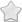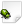OPEN  Crash report #1392  -  [D7C6CE88] ?? ()
Posted Sep 13, 2019 - updated Sep 13, 2019   Shortlink: http://arx.vg/1392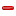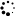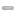0 Votes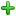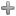Issue details
• Type of issue
Crash report
• Status
 New
• Assigned to
Not assigned to anyone
• Type of bug
Not triaged
• Likelihood
Not triaged
• Effect
Not triaged
• Posted by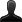CrashBot
• Owned by
Not owned by anyone
• Estimated time
Not estimated
• Category
Not determined
• Resolution
Not determined
• Priority
Not determined
• Reproducability
Not determined
• Severity
Not determined
• Targetted for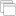Not determined
• OS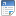Linux
• Architectureamd64
• Fixed inNot determined
Issue description
Illegal storage access: address not mapped to object

GDB stack trace: `[New LWP 20290][New LWP 20291][New LWP 20292][New LWP 20293][New LWP 20294][New LWP 20295][New LWP 20296][New LWP 20297][New LWP 20298][New LWP 20299][New LWP 20300][New LWP 20301][New LWP 20302][New LWP 20305][New LWP 20306][New LWP 20307][New LWP 20310][New LWP 30410][Thread debugging using libthread_db enabled]Using host libthread_db library "/lib/x86_64-linux-gnu/libthread_db.so.1".futex_wait_cancelable (private=<optimized out>, expected=0, futex_word=0x5619db5425b0) at ../sysdeps/unix/sysv/linux/futex-internal.h:88[Current thread is 1 (Thread 0x7fb9977c5800 (LWP 20289))] Id Target Id Frame * 1 Thread 0x7fb9977c5800 (LWP 20289) "arx" futex_wait_cancelable (private=<optimized out>, expected=0, futex_word=0x5619db5425b0) at ../sysdeps/unix/sysv/linux/futex-internal.h:88 2 Thread 0x7fb98f66f700 (LWP 20290) "arx:cs0" futex_wait_cancelable (private=<optimized out>, expected=0, futex_word=0x5619db104fc4) at ../sysdeps/unix/sysv/linux/futex-internal.h:88 3 Thread 0x7fb98ed0f700 (LWP 20291) "arx:disk\$0" futex_wait_cancelable (private=<optimized out>, expected=0, futex_word=0x5619db108ec8) at ../sysdeps/unix/sysv/linux/futex-internal.h:88 4 Thread 0x7fb98e50e700 (LWP 20292) "arx:sh0" futex_wait_cancelable (private=<optimized out>, expected=0, futex_word=0x5619db108570) at ../sysdeps/unix/sysv/linux/futex-internal.h:88 5 Thread 0x7fb98dd0d700 (LWP 20293) "arx:sh1" futex_wait_cancelable (private=<optimized out>, expected=0, futex_word=0x5619db108570) at ../sysdeps/unix/sysv/linux/futex-internal.h:88 6 Thread 0x7fb98d50c700 (LWP 20294) "arx:sh2" futex_wait_cancelable (private=<optimized out>, expected=0, futex_word=0x5619db108570) at ../sysdeps/unix/sysv/linux/futex-internal.h:88 7 Thread 0x7fb98cd0b700 (LWP 20295) "arx:sh3" futex_wait_cancelable (private=<optimized out>, expected=0, futex_word=0x5619db108570) at ../sysdeps/unix/sysv/linux/futex-internal.h:88 8 Thread 0x7fb977fff700 (LWP 20296) "arx:sh4" futex_wait_cancelable (private=<optimized out>, expected=0, futex_word=0x5619db108570) at ../sysdeps/unix/sysv/linux/futex-internal.h:88 9 Thread 0x7fb9777fe700 (LWP 20297) "arx:sh5" futex_wait_cancelable (private=<optimized out>, expected=0, futex_word=0x5619db108570) at ../sysdeps/unix/sysv/linux/futex-internal.h:88 10 Thread 0x7fb976ffd700 (LWP 20298) "arx:shlo0" futex_wait_cancelable (private=<optimized out>, expected=0, futex_word=0x5619db108af4) at ../sysdeps/unix/sysv/linux/futex-internal.h:88 11 Thread 0x7fb9767fc700 (LWP 20299) "arx:shlo1" futex_wait_cancelable (private=<optimized out>, expected=0, futex_word=0x5619db108af4) at ../sysdeps/unix/sysv/linux/futex-internal.h:88 12 Thread 0x7fb975ffb700 (LWP 20300) "arx:shlo2" futex_wait_cancelable (private=<optimized out>, expected=0, futex_word=0x5619db108af4) at ../sysdeps/unix/sysv/linux/futex-internal.h:88 13 Thread 0x7fb9757fa700 (LWP 20301) "arx:shlo3" futex_wait_cancelable (private=<optimized out>, expected=0, futex_word=0x5619db108af4) at ../sysdeps/unix/sysv/linux/futex-internal.h:88 14 Thread 0x7fb974ff9700 (LWP 20302) "arx:gdrv0" futex_wait_cancelable (private=<optimized out>, expected=0, futex_word=0x5619db498a1c) at ../sysdeps/unix/sysv/linux/futex-internal.h:88 15 Thread 0x7fb94ffff700 (LWP 20305) "threaded-ml" 0x00007fb9972b9729 in __GI___poll (fds=0x7fb954009c10, nfds=3, timeout=155) at ../sysdeps/unix/sysv/linux/poll.c:29 16 Thread 0x7fb974777700 (LWP 20306) "arx" futex_wait_cancelable (private=<optimized out>, expected=0, futex_word=0x5619db53755c) at ../sysdeps/unix/sysv/linux/futex-internal.h:88 17 Thread 0x7fb974576700 (LWP 20307) "arx" futex_abstimed_wait_cancelable (private=0, abstime=0x0, expected=0, futex_word=0x5619db058b20) at ../sysdeps/unix/sysv/linux/futex-internal.h:205 18 Thread 0x7fb93f7f7700 (LWP 20310) "Sound Update" 0x00005619d95fe005 in ?? () 19 Thread 0x7fb93e31a700 (LWP 30410) "Pathfinder" 0x00007fb997713440 in __GI___nanosleep (requested_time=0x7fb93e319ce0, remaining=0x0) at ../sysdeps/unix/sysv/linux/nanosleep.c:28 Thread 19 (Thread 0x7fb93e31a700 (LWP 30410)):#0 0x00007fb997713440 in __GI___nanosleep (requested_time=0x7fb93e319ce0, remaining=0x0) at ../sysdeps/unix/sysv/linux/nanosleep.c:28 resultvar = 18446744073709551100 sc_cancel_oldtype = 0 sc_ret = <optimized out>#1 0x00005619d95fbea1 in ?? ()No symbol table info available.#2 0x00005619d9428cfa in ?? ()No symbol table info available.#3 0x00005619d95fbe0a in ?? ()No symbol table info available.#4 0x00007fb997709182 in start_thread (arg=<optimized out>) at pthread_create.c:486 ret = <optimized out> pd = <optimized out> now = <optimized out> unwind_buf = {cancel_jmp_buf = {{jmp_buf = {140433589118720, -5494894612492445604, 140730067999550, 140730067999551, 0, 140433589116672, 5533879499083356252, 5534106556921157724}, mask_was_saved = 0}}, priv = {pad = {0x0, 0x0, 0x0, 0x0}, data = {prev = 0x0, cleanup = 0x0, canceltype = 0}}} not_first_call = <optimized out>#5 0x00007fb9972c5b1f in clone () at ../sysdeps/unix/sysv/linux/x86_64/clone.S:95No locals. Thread 18 (Thread 0x7fb93f7f7700 (LWP 20310)):#0 0x00005619d95fe005 in ?? ()No symbol table info available.#1 0x00005619d95fda89 in ?? ()No symbol table info available.#2 <signal handler called>No locals.#3 0x00005619d96c1c5c in ?? ()No symbol table info available.#4 0x00005619d944e76c in ?? ()No symbol table info available.#5 0x00005619d96cdd76 in ?? ()No symbol table info available.#6 0x00005619d95fbe0a in ?? ()No symbol table info available.#7 0x00007fb997709182 in start_thread (arg=<optimized out>) at pthread_create.c:486 ret = <optimized out> pd = <optimized out> now = <optimized out> unwind_buf = {cancel_jmp_buf = {{jmp_buf = {140433610995456, -5494894612492445604, 140730068013246, 140730068013247, 0, 140433610993408, 5533877792370727004, 5534106556921157724}, mask_was_saved = 0}}, priv = {pad = {0x0, 0x0, 0x0, 0x0}, data = {prev = 0x0, cleanup = 0x0, canceltype = 0}}} not_first_call = <optimized out>#8 0x00007fb9972c5b1f in clone () at ../sysdeps/unix/sysv/linux/x86_64/clone.S:95No locals. Thread 17 (Thread 0x7fb974576700 (LWP 20307)):#0 futex_abstimed_wait_cancelable (private=0, abstime=0x0, expected=0, futex_word=0x5619db058b20) at ../sysdeps/unix/sysv/linux/futex-internal.h:205 __ret = -512 oldtype = 0 err = <optimized out> oldtype = <optimized out> err = <optimized out> __ret = <optimized out> resultvar = <optimized out> __arg6 = <optimized out> __arg5 = <optimized out> __arg4 = <optimized out> __arg3 = <optimized out> __arg2 = <optimized out> __arg1 = <optimized out> _a6 = <optimized out> _a5 = <optimized out> _a4 = <optimized out> _a3 = <optimized out> _a2 = <optimized out> _a1 = <optimized out>#1 do_futex_wait (sem=sem@entry=0x5619db058b20, abstime=0x0) at sem_waitcommon.c:111 err = <optimized out>#2 0x00007fb997712178 in __new_sem_wait_slow (sem=0x5619db058b20, abstime=0x0) at sem_waitcommon.c:181 _buffer = {__routine = 0x7fb997712040 <__sem_wait_cleanup>, __arg = 0x5619db058b20, __canceltype = 0, __prev = 0x0} err = <optimized out> d = 0#3 0x00007fb997b4ffe9 in ?? () from /usr/lib/x86_64-linux-gnu/libopenal.so.1No symbol table info available.#4 0x00007fb997b152dd in ?? () from /usr/lib/x86_64-linux-gnu/libopenal.so.1No symbol table info available.#5 0x00007fb997b4fb57 in ?? () from /usr/lib/x86_64-linux-gnu/libopenal.so.1No symbol table info available.#6 0x00007fb997709182 in start_thread (arg=<optimized out>) at pthread_create.c:486 ret = <optimized out> pd = <optimized out> now = <optimized out> unwind_buf = {cancel_jmp_buf = {{jmp_buf = {140434497562368, -5494894612492445604, 140730068012222, 140730068012223, 140730068012368, 140434497560320, 5534043062175406172, 5534106556921157724}, mask_was_saved = 0}}, priv = {pad = {0x0, 0x0, 0x0, 0x0}, data = {prev = 0x0, cleanup = 0x0, canceltype = 0}}} not_first_call = <optimized out>#7 0x00007fb9972c5b1f in clone () at ../sysdeps/unix/sysv/linux/x86_64/clone.S:95No locals. Thread 16 (Thread 0x7fb974777700 (LWP 20306)):#0 futex_wait_cancelable (private=<optimized out>, expected=0, futex_word=0x5619db53755c) at ../sysdeps/unix/sysv/linux/futex-internal.h:88 __ret = -512 oldtype = 0 err = <optimized out> oldtype = <optimized out> err = <optimized out> __ret = <optimized out> resultvar = <optimized out> __arg4 = <optimized out> __arg3 = <optimized out> __arg2 = <optimized out> __arg1 = <optimized out> _a4 = <optimized out> _a3 = <optimized out> _a2 = <optimized out> _a1 = <optimized out>#1 __pthread_cond_wait_common (abstime=0x0, mutex=0x5619db541670, cond=0x5619db537530) at pthread_cond_wait.c:502 spin = 0 buffer = {__routine = 0x7fb99770f050 <__condvar_cleanup_waiting>, __arg = 0x7fb974776d60, __canceltype = 8192, __prev = 0x0} cbuffer = {wseq = 1462051, cond = 0x5619db537530, mutex = 0x5619db541670, private = 0} rt = <optimized out> err = <optimized out> g = 1 flags = <optimized out> g1_start = <optimized out> signals = <optimized out> result = 0 wseq = 1462051 seq = 731025 private = <optimized out> maxspin = <optimized out> err = <optimized out> result = <optimized out> wseq = <optimized out> g = <optimized out> seq = <optimized out> flags = <optimized out> private = <optimized out> signals = <optimized out> g1_start = <optimized out> spin = <optimized out> buffer = <optimized out> cbuffer = <optimized out> rt = <optimized out> s = <optimized out>#2 __pthread_cond_wait (cond=0x5619db537530, mutex=0x5619db541670) at pthread_cond_wait.c:655No locals.#3 0x00007fb996f2afb8 in pa_threaded_mainloop_wait () from /usr/lib/x86_64-linux-gnu/libpulse.so.0No symbol table info available.#4 0x00007fb997b49496 in ?? () from /usr/lib/x86_64-linux-gnu/libopenal.so.1No symbol table info available.#5 0x00007fb997b4fb57 in ?? () from /usr/lib/x86_64-linux-gnu/libopenal.so.1No symbol table info available.#6 0x00007fb997709182 in start_thread (arg=<optimized out>) at pthread_create.c:486 ret = <optimized out> pd = <optimized out> now = <optimized out> unwind_buf = {cancel_jmp_buf = {{jmp_buf = {140434499663616, -5494894612492445604, 140730068012030, 140730068012031, 140730068012176, 140434499661568, 5534042787834370140, 5534106556921157724}, mask_was_saved = 0}}, priv = {pad = {0x0, 0x0, 0x0, 0x0}, data = {prev = 0x0, cleanup = 0x0, canceltype = 0}}} not_first_call = <optimized out>#7 0x00007fb9972c5b1f in clone () at ../sysdeps/unix/sysv/linux/x86_64/clone.S:95No locals. Thread 15 (Thread 0x7fb94ffff700 (LWP 20305)):#0 0x00007fb9972b9729 in __GI___poll (fds=0x7fb954009c10, nfds=3, timeout=155) at ../sysdeps/unix/sysv/linux/poll.c:29 resultvar = 18446744073709551100 sc_cancel_oldtype = 0 sc_ret = <optimized out>#1 0x00007fb996f2a9f1 in ?? () from /usr/lib/x86_64-linux-gnu/libpulse.so.0No symbol table info available.#2 0x00007fb996f1c260 in pa_mainloop_poll () from /usr/lib/x86_64-linux-gnu/libpulse.so.0No symbol table info available.#3 0x00007fb996f1c8ae in pa_mainloop_iterate () from /usr/lib/x86_64-linux-gnu/libpulse.so.0No symbol table info available.#4 0x00007fb996f1c960 in pa_mainloop_run () from /usr/lib/x86_64-linux-gnu/libpulse.so.0No symbol table info available.#5 0x00007fb996f2a939 in ?? () from /usr/lib/x86_64-linux-gnu/libpulse.so.0No symbol table info available.#6 0x00007fb996a82788 in ?? () from /usr/lib/x86_64-linux-gnu/pulseaudio/libpulsecommon-12.2.soNo symbol table info available.#7 0x00007fb997709182 in start_thread (arg=<optimized out>) at pthread_create.c:486 ret = <optimized out> pd = <optimized out> now = <optimized out> unwind_buf = {cancel_jmp_buf = {{jmp_buf = {140433887852288, -5494894612492445604, 140730068012158, 140730068012159, 0, 140433887850240, 5533984449293588572, 5534106556921157724}, mask_was_saved = 0}}, priv = {pad = {0x0, 0x0, 0x0, 0x0}, data = {prev = 0x0, cleanup = 0x0, canceltype = 0}}} not_first_call = <optimized out>#8 0x00007fb9972c5b1f in clone () at ../sysdeps/unix/sysv/linux/x86_64/clone.S:95No locals. Thread 14 (Thread 0x7fb974ff9700 (LWP 20302)):#0 futex_wait_cancelable (private=<optimized out>, expected=0, futex_word=0x5619db498a1c) at ../sysdeps/unix/sysv/linux/futex-internal.h:88 __ret = -512 oldtype = 0 err = <optimized out> oldtype = <optimized out> err = <optimized out> __ret = <optimized out> resultvar = <optimized out> __arg4 = <optimized out> __arg3 = <optimized out> __arg2 = <optimized out> __arg1 = <optimized out> _a4 = <optimized out> _a3 = <optimized out> _a2 = <optimized out> _a1 = <optimized out>#1 __pthread_cond_wait_common (abstime=0x0, mutex=0x5619db4989c8, cond=0x5619db4989f0) at pthread_cond_wait.c:502 spin = 0 buffer = {__routine = 0x7fb99770f050 <__condvar_cleanup_waiting>, __arg = 0x7fb974ff8d40, __canceltype = -619775872, __prev = 0x0} cbuffer = {wseq = 17441899, cond = 0x5619db4989f0, mutex = 0x5619db4989c8, private = 0} rt = <optimized out> err = <optimized out> g = 1 flags = <optimized out> g1_start = <optimized out> signals = <optimized out> result = 0 wseq = 17441899 seq = 8720949 private = <optimized out> maxspin = <optimized out> err = <optimized out> result = <optimized out> wseq = <optimized out> g = <optimized out> seq = <optimized out> flags = <optimized out> private = <optimized out> signals = <optimized out> g1_start = <optimized out> spin = <optimized out> buffer = <optimized out> cbuffer = <optimized out> rt = <optimized out> s = <optimized out>#2 __pthread_cond_wait (cond=0x5619db4989f0, mutex=0x5619db4989c8) at pthread_cond_wait.c:655No locals.#3 0x00007fb993ad4f0b in ?? () from /usr/lib/x86_64-linux-gnu/dri/radeonsi_dri.soNo symbol table info available.#4 0x00007fb993ad4c77 in ?? () from /usr/lib/x86_64-linux-gnu/dri/radeonsi_dri.soNo symbol table info available.#5 0x00007fb997709182 in start_thread (arg=<optimized out>) at pthread_create.c:486 ret = <optimized out> pd = <optimized out> now = <optimized out> unwind_buf = {cancel_jmp_buf = {{jmp_buf = {140434508584704, -5494894612492445604, 140730068010350, 140730068010351, 0, 140434508582656, 5534043823995230300, 5534106556921157724}, mask_was_saved = 0}}, priv = {pad = {0x0, 0x0, 0x0, 0x0}, data = {prev = 0x0, cleanup = 0x0, canceltype = 0}}} not_first_call = <optimized out>#6 0x00007fb9972c5b1f in clone () at ../sysdeps/unix/sysv/linux/x86_64/clone.S:95No locals. Thread 13 (Thread 0x7fb9757fa700 (LWP 20301)):#0 futex_wait_cancelable (private=<optimized out>, expected=0, futex_word=0x5619db108af4) at ../sysdeps/unix/sysv/linux/futex-internal.h:88 __ret = -512 oldtype = 0 err = <optimized out> oldtype = <optimized out> err = <optimized out> __ret = <optimized out> resultvar = <optimized out> __arg4 = <optimized out> __arg3 = <optimized out> __arg2 = <optimized out> __arg1 = <optimized out> _a4 = <optimized out> _a3 = <optimized out> _a2 = <optimized out> _a1 = <optimized out>#1 __pthread_cond_wait_common (abstime=0x0, mutex=0x5619db108aa0, cond=0x5619db108ac8) at pthread_cond_wait.c:502 spin = 0 buffer = {__routine = 0x7fb99770f050 <__condvar_cleanup_waiting>, __arg = 0x7fb9757f9d40, __canceltype = 23040, __prev = 0x0} cbuffer = {wseq = 15, cond = 0x5619db108ac8, mutex = 0x5619db108aa0, private = 0} rt = <optimized out> err = <optimized out> g = 1 flags = <optimized out> g1_start = <optimized out> signals = <optimized out> result = 0 wseq = 15 seq = 7 private = <optimized out> maxspin = <optimized out> err = <optimized out> result = <optimized out> wseq = <optimized out> g = <optimized out> seq = <optimized out> flags = <optimized out> private = <optimized out> signals = <optimized out> g1_start = <optimized out> spin = <optimized out> buffer = <optimized out> cbuffer = <optimized out> rt = <optimized out> s = <optimized out>#2 __pthread_cond_wait (cond=0x5619db108ac8, mutex=0x5619db108aa0) at pthread_cond_wait.c:655No locals.#3 0x00007fb993ad4f0b in ?? () from /usr/lib/x86_64-linux-gnu/dri/radeonsi_dri.soNo symbol table info available.#4 0x00007fb993ad4c77 in ?? () from /usr/lib/x86_64-linux-gnu/dri/radeonsi_dri.soNo symbol table info available.#5 0x00007fb997709182 in start_thread (arg=<optimized out>) at pthread_create.c:486 ret = <optimized out> pd = <optimized out> now = <optimized out> unwind_buf = {cancel_jmp_buf = {{jmp_buf = {140434516977408, -5494894612492445604, 140730068007518, 140730068007519, 0, 140434516975360, 5534040525997217884, 5534106556921157724}, mask_was_saved = 0}}, priv = {pad = {0x0, 0x0, 0x0, 0x0}, data = {prev = 0x0, cleanup = 0x0, canceltype = 0}}} not_first_call = <optimized out>#6 0x00007fb9972c5b1f in clone () at ../sysdeps/unix/sysv/linux/x86_64/clone.S:95No locals. Thread 12 (Thread 0x7fb975ffb700 (LWP 20300)):#0 futex_wait_cancelable (private=<optimized out>, expected=0, futex_word=0x5619db108af4) at ../sysdeps/unix/sysv/linux/futex-internal.h:88 __ret = -512 oldtype = 0 err = <optimized out> oldtype = <optimized out> err = <optimized out> __ret = <optimized out> resultvar = <optimized out> __arg4 = <optimized out> __arg3 = <optimized out> __arg2 = <optimized out> __arg1 = <optimized out> _a4 = <optimized out> _a3 = <optimized out> _a2 = <optimized out> _a1 = <optimized out>#1 __pthread_cond_wait_common (abstime=0x0, mutex=0x5619db108aa0, cond=0x5619db108ac8) at pthread_cond_wait.c:502 spin = 0 buffer = {__routine = 0x7fb99770f050 <__condvar_cleanup_waiting>, __arg = 0x7fb975ffad40, __canceltype = 15872, __prev = 0x0} cbuffer = {wseq = 13, cond = 0x5619db108ac8, mutex = 0x5619db108aa0, private = 0} rt = <optimized out> err = <optimized out> g = 1 flags = <optimized out> g1_start = <optimized out> signals = <optimized out> result = 0 wseq = 13 seq = 6 private = <optimized out> maxspin = <optimized out> err = <optimized out> result = <optimized out> wseq = <optimized out> g = <optimized out> seq = <optimized out> flags = <optimized out> private = <optimized out> signals = <optimized out> g1_start = <optimized out> spin = <optimized out> buffer = <optimized out> cbuffer = <optimized out> rt = <optimized out> s = <optimized out>#2 __pthread_cond_wait (cond=0x5619db108ac8, mutex=0x5619db108aa0) at pthread_cond_wait.c:655No locals.#3 0x00007fb993ad4f0b in ?? () from /usr/lib/x86_64-linux-gnu/dri/radeonsi_dri.soNo symbol table info available.#4 0x00007fb993ad4c77 in ?? () from /usr/lib/x86_64-linux-gnu/dri/radeonsi_dri.soNo symbol table info available.#5 0x00007fb997709182 in start_thread (arg=<optimized out>) at pthread_create.c:486 ret = <optimized out> pd = <optimized out> now = <optimized out> unwind_buf = {cancel_jmp_buf = {{jmp_buf = {140434525370112, -5494894612492445604, 140730068007518, 140730068007519, 0, 140434525368064, 5534041626045716572, 5534106556921157724}, mask_was_saved = 0}}, priv = {pad = {0x0, 0x0, 0x0, 0x0}, data = {prev = 0x0, cleanup = 0x0, canceltype = 0}}} not_first_call = <optimized out>#6 0x00007fb9972c5b1f in clone () at ../sysdeps/unix/sysv/linux/x86_64/clone.S:95No locals. Thread 11 (Thread 0x7fb9767fc700 (LWP 20299)):#0 futex_wait_cancelable (private=<optimized out>, expected=0, futex_word=0x5619db108af4) at ../sysdeps/unix/sysv/linux/futex-internal.h:88 __ret = -512 oldtype = 0 err = <optimized out> oldtype = <optimized out> err = <optimized out> __ret = <optimized out> resultvar = <optimized out> __arg4 = <optimized out> __arg3 = <optimized out> __arg2 = <optimized out> __arg1 = <optimized out> _a4 = <optimized out> _a3 = <optimized out> _a2 = <optimized out> _a1 = <optimized out>#1 __pthread_cond_wait_common (abstime=0x0, mutex=0x5619db108aa0, cond=0x5619db108ac8) at pthread_cond_wait.c:502 spin = 0 buffer = {__routine = 0x7fb99770f050 <__condvar_cleanup_waiting>, __arg = 0x7fb9767fbd40, __canceltype = 11776, __prev = 0x0} cbuffer = {wseq = 11, cond = 0x5619db108ac8, mutex = 0x5619db108aa0, private = 0} rt = <optimized out> err = <optimized out> g = 1 flags = <optimized out> g1_start = <optimized out> signals = <optimized out> result = 0 wseq = 11 seq = 5 private = <optimized out> maxspin = <optimized out> err = <optimized out> result = <optimized out> wseq = <optimized out> g = <optimized out> seq = <optimized out> flags = <optimized out> private = <optimized out> signals = <optimized out> g1_start = <optimized out> spin = <optimized out> buffer = <optimized out> cbuffer = <optimized out> rt = <optimized out> s = <optimized out>#2 __pthread_cond_wait (cond=0x5619db108ac8, mutex=0x5619db108aa0) at pthread_cond_wait.c:655No locals.#3 0x00007fb993ad4f0b in ?? () from /usr/lib/x86_64-linux-gnu/dri/radeonsi_dri.soNo symbol table info available.#4 0x00007fb993ad4c77 in ?? () from /usr/lib/x86_64-linux-gnu/dri/radeonsi_dri.soNo symbol table info available.#5 0x00007fb997709182 in start_thread (arg=<optimized out>) at pthread_create.c:486 ret = <optimized out> pd = <optimized out> now = <optimized out> unwind_buf = {cancel_jmp_buf = {{jmp_buf = {140434533762816, -5494894612492445604, 140730068007518, 140730068007519, 0, 140434533760768, 5534038328047704156, 5534106556921157724}, mask_was_saved = 0}}, priv = {pad = {0x0, 0x0, 0x0, 0x0}, data = {prev = 0x0, cleanup = 0x0, canceltype = 0}}} not_first_call = <optimized out>#6 0x00007fb9972c5b1f in clone () at ../sysdeps/unix/sysv/linux/x86_64/clone.S:95No locals. Thread 10 (Thread 0x7fb976ffd700 (LWP 20298)):#0 futex_wait_cancelable (private=<optimized out>, expected=0, futex_word=0x5619db108af4) at ../sysdeps/unix/sysv/linux/futex-internal.h:88 __ret = -512 oldtype = 0 err = <optimized out> oldtype = <optimized out> err = <optimized out> __ret = <optimized out> resultvar = <optimized out> __arg4 = <optimized out> __arg3 = <optimized out> __arg2 = <optimized out> __arg1 = <optimized out> _a4 = <optimized out> _a3 = <optimized out> _a2 = <optimized out> _a1 = <optimized out>#1 __pthread_cond_wait_common (abstime=0x0, mutex=0x5619db108aa0, cond=0x5619db108ac8) at pthread_cond_wait.c:502 spin = 0 buffer = {__routine = 0x7fb99770f050 <__condvar_cleanup_waiting>, __arg = 0x7fb976ffcd40, __canceltype = 7680, __prev = 0x0} cbuffer = {wseq = 9, cond = 0x5619db108ac8, mutex = 0x5619db108aa0, private = 0} rt = <optimized out> err = <optimized out> g = 1 flags = <optimized out> g1_start = <optimized out> signals = <optimized out> result = 0 wseq = 9 seq = 4 private = <optimized out> maxspin = <optimized out> err = <optimized out> result = <optimized out> wseq = <optimized out> g = <optimized out> seq = <optimized out> flags = <optimized out> private = <optimized out> signals = <optimized out> g1_start = <optimized out> spin = <optimized out> buffer = <optimized out> cbuffer = <optimized out> rt = <optimized out> s = <optimized out>#2 __pthread_cond_wait (cond=0x5619db108ac8, mutex=0x5619db108aa0) at pthread_cond_wait.c:655No locals.#3 0x00007fb993ad4f0b in ?? () from /usr/lib/x86_64-linux-gnu/dri/radeonsi_dri.soNo symbol table info available.#4 0x00007fb993ad4c77 in ?? () from /usr/lib/x86_64-linux-gnu/dri/radeonsi_dri.soNo symbol table info available.#5 0x00007fb997709182 in start_thread (arg=<optimized out>) at pthread_create.c:486 ret = <optimized out> pd = <optimized out> now = <optimized out> unwind_buf = {cancel_jmp_buf = {{jmp_buf = {140434542155520, -5494894612492445604, 140730068007518, 140730068007519, 0, 140434542153472, 5534039423801235548, 5534106556921157724}, mask_was_saved = 0}}, priv = {pad = {0x0, 0x0, 0x0, 0x0}, data = {prev = 0x0, cleanup = 0x0, canceltype = 0}}} not_first_call = <optimized out>#6 0x00007fb9972c5b1f in clone () at ../sysdeps/unix/sysv/linux/x86_64/clone.S:95No locals. Thread 9 (Thread 0x7fb9777fe700 (LWP 20297)):#0 futex_wait_cancelable (private=<optimized out>, expected=0, futex_word=0x5619db108570) at ../sysdeps/unix/sysv/linux/futex-internal.h:88 __ret = -512 oldtype = 0 err = <optimized out> oldtype = <optimized out> err = <optimized out> __ret = <optimized out> resultvar = <optimized out> __arg4 = <optimized out> __arg3 = <optimized out> __arg2 = <optimized out> __arg1 = <optimized out> _a4 = <optimized out> _a3 = <optimized out> _a2 = <optimized out> _a1 = <optimized out>#1 __pthread_cond_wait_common (abstime=0x0, mutex=0x5619db108520, cond=0x5619db108548) at pthread_cond_wait.c:502 spin = 0 buffer = {__routine = 0x7fb99770f050 <__condvar_cleanup_waiting>, __arg = 0x7fb9777fdd40, __canceltype = -523527488, __prev = 0x0} cbuffer = {wseq = 70, cond = 0x5619db108548, mutex = 0x5619db108520, private = 0} rt = <optimized out> err = <optimized out> g = 0 flags = <optimized out> g1_start = <optimized out> signals = <optimized out> result = 0 wseq = 70 seq = 35 private = <optimized out> maxspin = <optimized out> err = <optimized out> result = <optimized out> wseq = <optimized out> g = <optimized out> seq = <optimized out> flags = <optimized out> private = <optimized out> signals = <optimized out> g1_start = <optimized out> spin = <optimized out> buffer = <optimized out> cbuffer = <optimized out> rt = <optimized out> s = <optimized out>#2 __pthread_cond_wait (cond=0x5619db108548, mutex=0x5619db108520) at pthread_cond_wait.c:655No locals.#3 0x00007fb993ad4f0b in ?? () from /usr/lib/x86_64-linux-gnu/dri/radeonsi_dri.soNo symbol table info available.#4 0x00007fb993ad4c77 in ?? () from /usr/lib/x86_64-linux-gnu/dri/radeonsi_dri.soNo symbol table info available.#5 0x00007fb997709182 in start_thread (arg=<optimized out>) at pthread_create.c:486 ret = <optimized out> pd = <optimized out> now = <optimized out> unwind_buf = {cancel_jmp_buf = {{jmp_buf = {140434550548224, -5494894612492445604, 140730068007518, 140730068007519, 0, 140434550546176, 5534036125803223132, 5534106556921157724}, mask_was_saved = 0}}, priv = {pad = {0x0, 0x0, 0x0, 0x0}, data = {prev = 0x0, cleanup = 0x0, canceltype = 0}}} not_first_call = <optimized out>#6 0x00007fb9972c5b1f in clone () at ../sysdeps/unix/sysv/linux/x86_64/clone.S:95No locals. Thread 8 (Thread 0x7fb977fff700 (LWP 20296)):#0 futex_wait_cancelable (private=<optimized out>, expected=0, futex_word=0x5619db108570) at ../sysdeps/unix/sysv/linux/futex-internal.h:88 __ret = -512 oldtype = 0 err = <optimized out> oldtype = <optimized out> err = <optimized out> __ret = <optimized out> resultvar = <optimized out> __arg4 = <optimized out> __arg3 = <optimized out> __arg2 = <optimized out> __arg1 = <optimized out> _a4 = <optimized out> _a3 = <optimized out> _a2 = <optimized out> _a1 = <optimized out>#1 __pthread_cond_wait_common (abstime=0x0, mutex=0x5619db108520, cond=0x5619db108548) at pthread_cond_wait.c:502 spin = 0 buffer = {__routine = 0x7fb99770f050 <__condvar_cleanup_waiting>, __arg = 0x7fb977ffed40, __canceltype = -568391824, __prev = 0x0} cbuffer = {wseq = 78, cond = 0x5619db108548, mutex = 0x5619db108520, private = 0} rt = <optimized out> err = <optimized out> g = 0 flags = <optimized out> g1_start = <optimized out> signals = <optimized out> result = 0 wseq = 78 seq = 39 private = <optimized out> maxspin = <optimized out> err = <optimized out> result = <optimized out> wseq = <optimized out> g = <optimized out> seq = <optimized out> flags = <optimized out> private = <optimized out> signals = <optimized out> g1_start = <optimized out> spin = <optimized out> buffer = <optimized out> cbuffer = <optimized out> rt = <optimized out> s = <optimized out>#2 __pthread_cond_wait (cond=0x5619db108548, mutex=0x5619db108520) at pthread_cond_wait.c:655No locals.#3 0x00007fb993ad4f0b in ?? () from /usr/lib/x86_64-linux-gnu/dri/radeonsi_dri.soNo symbol table info available.#4 0x00007fb993ad4c77 in ?? () from /usr/lib/x86_64-linux-gnu/dri/radeonsi_dri.soNo symbol table info available.#5 0x00007fb997709182 in start_thread (arg=<optimized out>) at pthread_create.c:486 ret = <optimized out> pd = <optimized out> now = <optimized out> unwind_buf = {cancel_jmp_buf = {{jmp_buf = {140434558940928, -5494894612492445604, 140730068007518, 140730068007519, 0, 140434558938880, 5534037225851721820, 5534106556921157724}, mask_was_saved = 0}}, priv = {pad = {0x0, 0x0, 0x0, 0x0}, data = {prev = 0x0, cleanup = 0x0, canceltype = 0}}} not_first_call = <optimized out>#6 0x00007fb9972c5b1f in clone () at ../sysdeps/unix/sysv/linux/x86_64/clone.S:95No locals. Thread 7 (Thread 0x7fb98cd0b700 (LWP 20295)):#0 futex_wait_cancelable (private=<optimized out>, expected=0, futex_word=0x5619db108570) at ../sysdeps/unix/sysv/linux/futex-internal.h:88 __ret = -512 oldtype = 0 err = <optimized out> oldtype = <optimized out> err = <optimized out> __ret = <optimized out> resultvar = <optimized out> __arg4 = <optimized out> __arg3 = <optimized out> __arg2 = <optimized out> __arg1 = <optimized out> _a4 = <optimized out> _a3 = <optimized out> _a2 = <optimized out> _a1 = <optimized out>#1 __pthread_cond_wait_common (abstime=0x0, mutex=0x5619db108520, cond=0x5619db108548) at pthread_cond_wait.c:502 spin = 0 buffer = {__routine = 0x7fb99770f050 <__condvar_cleanup_waiting>, __arg = 0x7fb98cd0ad40, __canceltype = -496606480, __prev = 0x0} cbuffer = {wseq = 80, cond = 0x5619db108548, mutex = 0x5619db108520, private = 0} rt = <optimized out> err = <optimized out> g = 0 flags = <optimized out> g1_start = <optimized out> signals = <optimized out> result = 0 wseq = 80 seq = 40 private = <optimized out> maxspin = <optimized out> err = <optimized out> result = <optimized out> wseq = <optimized out> g = <optimized out> seq = <optimized out> flags = <optimized out> private = <optimized out> signals = <optimized out> g1_start = <optimized out> spin = <optimized out> buffer = <optimized out> cbuffer = <optimized out> rt = <optimized out> s = <optimized out>#2 __pthread_cond_wait (cond=0x5619db108548, mutex=0x5619db108520) at pthread_cond_wait.c:655No locals.#3 0x00007fb993ad4f0b in ?? () from /usr/lib/x86_64-linux-gnu/dri/radeonsi_dri.soNo symbol table info available.#4 0x00007fb993ad4c77 in ?? () from /usr/lib/x86_64-linux-gnu/dri/radeonsi_dri.soNo symbol table info available.#5 0x00007fb997709182 in start_thread (arg=<optimized out>) at pthread_create.c:486 ret = <optimized out> pd = <optimized out> now = <optimized out> unwind_buf = {cancel_jmp_buf = {{jmp_buf = {140434908165888, -5494894612492445604, 140730068007518, 140730068007519, 0, 140434908163840, 5534132121006643292, 5534106556921157724}, mask_was_saved = 0}}, priv = {pad = {0x0, 0x0, 0x0, 0x0}, data = {prev = 0x0, cleanup = 0x0, canceltype = 0}}} not_first_call = <optimized out>#6 0x00007fb9972c5b1f in clone () at ../sysdeps/unix/sysv/linux/x86_64/clone.S:95No locals. Thread 6 (Thread 0x7fb98d50c700 (LWP 20294)):#0 futex_wait_cancelable (private=<optimized out>, expected=0, futex_word=0x5619db108570) at ../sysdeps/unix/sysv/linux/futex-internal.h:88 __ret = -512 oldtype = 0 err = <optimized out> oldtype = <optimized out> err = <optimized out> __ret = <optimized out> resultvar = <optimized out> __arg4 = <optimized out> __arg3 = <optimized out> __arg2 = <optimized out> __arg1 = <optimized out> _a4 = <optimized out> _a3 = <optimized out> _a2 = <optimized out> _a1 = <optimized out>#1 __pthread_cond_wait_common (abstime=0x0, mutex=0x5619db108520, cond=0x5619db108548) at pthread_cond_wait.c:502 spin = 0 buffer = {__routine = 0x7fb99770f050 <__condvar_cleanup_waiting>, __arg = 0x7fb98d50bd40, __canceltype = -508304576, __prev = 0x0} cbuffer = {wseq = 74, cond = 0x5619db108548, mutex = 0x5619db108520, private = 0} rt = <optimized out> err = <optimized out> g = 0 flags = <optimized out> g1_start = <optimized out> signals = <optimized out> result = 0 wseq = 74 seq = 37 private = <optimized out> maxspin = <optimized out> err = <optimized out> result = <optimized out> wseq = <optimized out> g = <optimized out> seq = <optimized out> flags = <optimized out> private = <optimized out> signals = <optimized out> g1_start = <optimized out> spin = <optimized out> buffer = <optimized out> cbuffer = <optimized out> rt = <optimized out> s = <optimized out>#2 __pthread_cond_wait (cond=0x5619db108548, mutex=0x5619db108520) at pthread_cond_wait.c:655No locals.#3 0x00007fb993ad4f0b in ?? () from /usr/lib/x86_64-linux-gnu/dri/radeonsi_dri.soNo symbol table info available.#4 0x00007fb993ad4c77 in ?? () from /usr/lib/x86_64-linux-gnu/dri/radeonsi_dri.soNo symbol table info available.#5 0x00007fb997709182 in start_thread (arg=<optimized out>) at pthread_create.c:486 ret = <optimized out> pd = <optimized out> now = <optimized out> unwind_buf = {cancel_jmp_buf = {{jmp_buf = {140434916558592, -5494894612492445604, 140730068007518, 140730068007519, 0, 140434916556544, 5534128823008630876, 5534106556921157724}, mask_was_saved = 0}}, priv = {pad = {0x0, 0x0, 0x0, 0x0}, data = {prev = 0x0, cleanup = 0x0, canceltype = 0}}} not_first_call = <optimized out>#6 0x00007fb9972c5b1f in clone () at ../sysdeps/unix/sysv/linux/x86_64/clone.S:95No locals. Thread 5 (Thread 0x7fb98dd0d700 (LWP 20293)):#0 futex_wait_cancelable (private=<optimized out>, expected=0, futex_word=0x5619db108570) at ../sysdeps/unix/sysv/linux/futex-internal.h:88 __ret = -512 oldtype = 0 err = <optimized out> oldtype = <optimized out> err = <optimized out> __ret = <optimized out> resultvar = <optimized out> __arg4 = <optimized out> __arg3 = <optimized out> __arg2 = <optimized out> __arg1 = <optimized out> _a4 = <optimized out> _a3 = <optimized out> _a2 = <optimized out> _a1 = <optimized out>#1 __pthread_cond_wait_common (abstime=0x0, mutex=0x5619db108520, cond=0x5619db108548) at pthread_cond_wait.c:502 spin = 0 buffer = {__routine = 0x7fb99770f050 <__condvar_cleanup_waiting>, __arg = 0x7fb98dd0cd40, __canceltype = -498838368, __prev = 0x0} cbuffer = {wseq = 76, cond = 0x5619db108548, mutex = 0x5619db108520, private = 0} rt = <optimized out> err = <optimized out> g = 0 flags = <optimized out> g1_start = <optimized out> signals = <optimized out> result = 0 wseq = 76 seq = 38 private = <optimized out> maxspin = <optimized out> err = <optimized out> result = <optimized out> wseq = <optimized out> g = <optimized out> seq = <optimized out> flags = <optimized out> private = <optimized out> signals = <optimized out> g1_start = <optimized out> spin = <optimized out> buffer = <optimized out> cbuffer = <optimized out> rt = <optimized out> s = <optimized out>#2 __pthread_cond_wait (cond=0x5619db108548, mutex=0x5619db108520) at pthread_cond_wait.c:655No locals.#3 0x00007fb993ad4f0b in ?? () from /usr/lib/x86_64-linux-gnu/dri/radeonsi_dri.soNo symbol table info available.#4 0x00007fb993ad4c77 in ?? () from /usr/lib/x86_64-linux-gnu/dri/radeonsi_dri.soNo symbol table info available.#5 0x00007fb997709182 in start_thread (arg=<optimized out>) at pthread_create.c:486 ret = <optimized out> pd = <optimized out> now = <optimized out> unwind_buf = {cancel_jmp_buf = {{jmp_buf = {140434924951296, -5494894612492445604, 140730068007518, 140730068007519, 0, 140434924949248, 5534129918762162268, 5534106556921157724}, mask_was_saved = 0}}, priv = {pad = {0x0, 0x0, 0x0, 0x0}, data = {prev = 0x0, cleanup = 0x0, canceltype = 0}}} not_first_call = <optimized out>#6 0x00007fb9972c5b1f in clone () at ../sysdeps/unix/sysv/linux/x86_64/clone.S:95No locals. Thread 4 (Thread 0x7fb98e50e700 (LWP 20292)):#0 futex_wait_cancelable (private=<optimized out>, expected=0, futex_word=0x5619db108570) at ../sysdeps/unix/sysv/linux/futex-internal.h:88 __ret = -512 oldtype = 0 err = <optimized out> oldtype = <optimized out> err = <optimized out> __ret = <optimized out> resultvar = <optimized out> __arg4 = <optimized out> __arg3 = <optimized out> __arg2 = <optimized out> __arg1 = <optimized out> _a4 = <optimized out> _a3 = <optimized out> _a2 = <optimized out> _a1 = <optimized out>#1 __pthread_cond_wait_common (abstime=0x0, mutex=0x5619db108520, cond=0x5619db108548) at pthread_cond_wait.c:502 spin = 0 buffer = {__routine = 0x7fb99770f050 <__condvar_cleanup_waiting>, __arg = 0x7fb98e50dd40, __canceltype = -468455184, __prev = 0x0} cbuffer = {wseq = 72, cond = 0x5619db108548, mutex = 0x5619db108520, private = 0} rt = <optimized out> err = <optimized out> g = 0 flags = <optimized out> g1_start = <optimized out> signals = <optimized out> result = 0 wseq = 72 seq = 36 private = <optimized out> maxspin = <optimized out> err = <optimized out> result = <optimized out> wseq = <optimized out> g = <optimized out> seq = <optimized out> flags = <optimized out> private = <optimized out> signals = <optimized out> g1_start = <optimized out> spin = <optimized out> buffer = <optimized out> cbuffer = <optimized out> rt = <optimized out> s = <optimized out>#2 __pthread_cond_wait (cond=0x5619db108548, mutex=0x5619db108520) at pthread_cond_wait.c:655No locals.#3 0x00007fb993ad4f0b in ?? () from /usr/lib/x86_64-linux-gnu/dri/radeonsi_dri.soNo symbol table info available.#4 0x00007fb993ad4c77 in ?? () from /usr/lib/x86_64-linux-gnu/dri/radeonsi_dri.soNo symbol table info available.#5 0x00007fb997709182 in start_thread (arg=<optimized out>) at pthread_create.c:486 ret = <optimized out> pd = <optimized out> now = <optimized out> unwind_buf = {cancel_jmp_buf = {{jmp_buf = {140434933344000, -5494894612492445604, 140730068007518, 140730068007519, 0, 140434933341952, 5534126620764149852, 5534106556921157724}, mask_was_saved = 0}}, priv = {pad = {0x0, 0x0, 0x0, 0x0}, data = {prev = 0x0, cleanup = 0x0, canceltype = 0}}} not_first_call = <optimized out>#6 0x00007fb9972c5b1f in clone () at ../sysdeps/unix/sysv/linux/x86_64/clone.S:95No locals. Thread 3 (Thread 0x7fb98ed0f700 (LWP 20291)):#0 futex_wait_cancelable (private=<optimized out>, expected=0, futex_word=0x5619db108ec8) at ../sysdeps/unix/sysv/linux/futex-internal.h:88 __ret = -512 oldtype = 0 err = <optimized out> oldtype = <optimized out> err = <optimized out> __ret = <optimized out> resultvar = <optimized out> __arg4 = <optimized out> __arg3 = <optimized out> __arg2 = <optimized out> __arg1 = <optimized out> _a4 = <optimized out> _a3 = <optimized out> _a2 = <optimized out> _a1 = <optimized out>#1 __pthread_cond_wait_common (abstime=0x0, mutex=0x5619db108e78, cond=0x5619db108ea0) at pthread_cond_wait.c:502 spin = 0 buffer = {__routine = 0x7fb99770f050 <__condvar_cleanup_waiting>, __arg = 0x7fb98ed0ed40, __canceltype = 35, __prev = 0x0} cbuffer = {wseq = 0, cond = 0x5619db108ea0, mutex = 0x5619db108e78, private = 0} rt = <optimized out> err = <optimized out> g = 0 flags = <optimized out> g1_start = <optimized out> signals = <optimized out> result = 0 wseq = 0 seq = 0 private = <optimized out> maxspin = <optimized out> err = <optimized out> result = <optimized out> wseq = <optimized out> g = <optimized out> seq = <optimized out> flags = <optimized out> private = <optimized out> signals = <optimized out> g1_start = <optimized out> spin = <optimized out> buffer = <optimized out> cbuffer = <optimized out> rt = <optimized out> s = <optimized out>#2 __pthread_cond_wait (cond=0x5619db108ea0, mutex=0x5619db108e78) at pthread_cond_wait.c:655No locals.#3 0x00007fb993ad4f0b in ?? () from /usr/lib/x86_64-linux-gnu/dri/radeonsi_dri.soNo symbol table info available.#4 0x00007fb993ad4c77 in ?? () from /usr/lib/x86_64-linux-gnu/dri/radeonsi_dri.soNo symbol table info available.#5 0x00007fb997709182 in start_thread (arg=<optimized out>) at pthread_create.c:486 ret = <optimized out> pd = <optimized out> now = <optimized out> unwind_buf = {cancel_jmp_buf = {{jmp_buf = {140434941736704, -5494894612492445604, 140730068006766, 140730068006767, 0, 140434941734656, 5534127720812648540, 5534106556921157724}, mask_was_saved = 0}}, priv = {pad = {0x0, 0x0, 0x0, 0x0}, data = {prev = 0x0, cleanup = 0x0, canceltype = 0}}} not_first_call = <optimized out>#6 0x00007fb9972c5b1f in clone () at ../sysdeps/unix/sysv/linux/x86_64/clone.S:95No locals. Thread 2 (Thread 0x7fb98f66f700 (LWP 20290)):#0 futex_wait_cancelable (private=<optimized out>, expected=0, futex_word=0x5619db104fc4) at ../sysdeps/unix/sysv/linux/futex-internal.h:88 __ret = -512 oldtype = 0 err = <optimized out> oldtype = <optimized out> err = <optimized out> __ret = <optimized out> resultvar = <optimized out> __arg4 = <optimized out> __arg3 = <optimized out> __arg2 = <optimized out> __arg1 = <optimized out> _a4 = <optimized out> _a3 = <optimized out> _a2 = <optimized out> _a1 = <optimized out>#1 __pthread_cond_wait_common (abstime=0x0, mutex=0x5619db104f70, cond=0x5619db104f98) at pthread_cond_wait.c:502 spin = 0 buffer = {__routine = 0x7fb99770f050 <__condvar_cleanup_waiting>, __arg = 0x7fb98f66ed40, __canceltype = 6, __prev = 0x0} cbuffer = {wseq = 3995255, cond = 0x5619db104f98, mutex = 0x5619db104f70, private = 0} rt = <optimized out> err = <optimized out> g = 1 flags = <optimized out> g1_start = <optimized out> signals = <optimized out> result = 0 wseq = 3995255 seq = 1997627 private = <optimized out> maxspin = <optimized out> err = <optimized out> result = <optimized out> wseq = <optimized out> g = <optimized out> seq = <optimized out> flags = <optimized out> private = <optimized out> signals = <optimized out> g1_start = <optimized out> spin = <optimized out> buffer = <optimized out> cbuffer = <optimized out> rt = <optimized out> s = <optimized out>#2 __pthread_cond_wait (cond=0x5619db104f98, mutex=0x5619db104f70) at pthread_cond_wait.c:655No locals.#3 0x00007fb993ad4f0b in ?? () from /usr/lib/x86_64-linux-gnu/dri/radeonsi_dri.soNo symbol table info available.#4 0x00007fb993ad4c77 in ?? () from /usr/lib/x86_64-linux-gnu/dri/radeonsi_dri.soNo symbol table info available.#5 0x00007fb997709182 in start_thread (arg=<optimized out>) at pthread_create.c:486 ret = <optimized out> pd = <optimized out> now = <optimized out> unwind_buf = {cancel_jmp_buf = {{jmp_buf = {140434951567104, -5494894612492445604, 140730068007662, 140730068007663, 0, 140434951565056, 5534124302018680924, 5534106556921157724}, mask_was_saved = 0}}, priv = {pad = {0x0, 0x0, 0x0, 0x0}, data = {prev = 0x0, cleanup = 0x0, canceltype = 0}}} not_first_call = <optimized out>#6 0x00007fb9972c5b1f in clone () at ../sysdeps/unix/sysv/linux/x86_64/clone.S:95No locals. Thread 1 (Thread 0x7fb9977c5800 (LWP 20289)):#0 futex_wait_cancelable (private=<optimized out>, expected=0, futex_word=0x5619db5425b0) at ../sysdeps/unix/sysv/linux/futex-internal.h:88 __ret = -512 oldtype = 0 err = <optimized out> oldtype = <optimized out> err = <optimized out> __ret = <optimized out> resultvar = <optimized out> __arg4 = <optimized out> __arg3 = <optimized out> __arg2 = <optimized out> __arg1 = <optimized out> _a4 = <optimized out> _a3 = <optimized out> _a2 = <optimized out> _a1 = <optimized out>#1 __pthread_cond_wait_common (abstime=0x0, mutex=0x5619db542560, cond=0x5619db542588) at pthread_cond_wait.c:502 spin = 0 buffer = {__routine = 0x7fb99770f050 <__condvar_cleanup_waiting>, __arg = 0x7ffe45b695b0, __canceltype = 1169593920, __prev = 0x0} cbuffer = {wseq = 16668, cond = 0x5619db542588, mutex = 0x5619db542560, private = 0} rt = <optimized out> err = <optimized out> g = 0 flags = <optimized out> g1_start = <optimized out> signals = <optimized out> result = 0 wseq = 16668 seq = 8334 private = <optimized out> maxspin = <optimized out> err = <optimized out> result = <optimized out> wseq = <optimized out> g = <optimized out> seq = <optimized out> flags = <optimized out> private = <optimized out> signals = <optimized out> g1_start = <optimized out> spin = <optimized out> buffer = <optimized out> cbuffer = <optimized out> rt = <optimized out> s = <optimized out>#2 __pthread_cond_wait (cond=0x5619db542588, mutex=0x5619db542560) at pthread_cond_wait.c:655No locals.#3 0x00005619d95fa3ca in ?? ()No symbol table info available.#4 0x00005619d96bf728 in ?? ()No symbol table info available.#5 0x00005619d944f402 in ?? ()No symbol table info available.#6 0x00005619d960e96d in ?? ()No symbol table info available.#7 0x00005619d9467885 in ?? ()No symbol table info available.#8 0x00005619d946261a in ?? ()No symbol table info available.#9 0x00005619d94625b2 in ?? ()No symbol table info available.#10 0x00005619d946c87b in ?? ()No symbol table info available.#11 0x00005619d9485208 in ?? ()No symbol table info available.#12 0x00007fb9971ceb6b in __libc_start_main (main=0x5619d9484dd5, argc=1, argv=0x7ffe45b69d38, init=<optimized out>, fini=<optimized out>, rtld_fini=<optimized out>, stack_end=0x7ffe45b69d28) at ../csu/libc-start.c:308 self = <optimized out> result = <optimized out> unwind_buf = {cancel_jmp_buf = {{jmp_buf = {0, 2273619176372181084, 94669019180224, 140730068016432, 0, 0, 5495162421841369180, 5534106245870917724}, mask_was_saved = 0}}, priv = {pad = {0x0, 0x0, 0x7ffe45b69d48, 0x7fb997d2c190}, data = {prev = 0x0, cleanup = 0x0, canceltype = 1169595720}}} not_first_call = <optimized out>#13 0x00005619d9426cea in _start ()No symbol table info available.[Inferior 1 (process 20289) detached]`

Steps to reproduce this issue
Nothing entered.

#1
Comment posted byCrashBot
Sep 13, 12:18
A file was uploaded. arx.log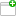This comment was attached:

arxcrash-20289-2501430790
#3
Comment posted byCrashBot
Sep 13, 12:18
A file was uploaded. cfg.iniThis comment was attached:

arxcrash-20289-2501430790
#4
Comment posted byCrashBot
Sep 13, 12:18
A file was uploaded. crash.xmlThis comment was attached:

arxcrash-20289-2501430790